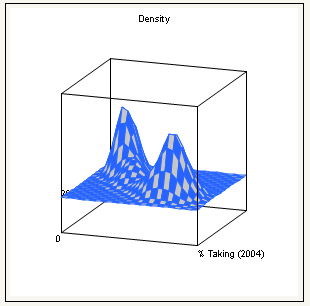Basic Analysis > Bivariate Analysis > Fitting Menus > Fitting Menu Options
Publication date: 07/30/2020

The Fitting menu options depend on the selected fit.

### Options That Apply to Most Fits

Line of Fit

Displays or hides the line or curve describing the model fit. For the Bivariate Normal Ellipse report, this option shows or hides the ellipse representing the contour border. Not applicable for Quantile Density Contours.

Line Color

Lets you select from a palette of colors for assigning a color to each fit. Not applicable for Quantile Density Contours.

Line Style

Lets you select from the palette of line styles for each fit. Not applicable for Quantile Density Contours.

Line Width

Lets you change the line widths for the line of fit. The default line width is the thinnest line. Not applicable for Quantile Density Contours.

Report

Turns the fit’s report on and off. Does not modify the Bivariate plot.

Remove Fit

Removes the fit from the graph and removes its report.

### Options That Apply to Multiple Fits

Confid Curves Fit

Displays or hides the confidence limits for the expected value (mean). This option is not available for the Fit Spline, Density Ellipse, Fit Each Value, and Fit Orthogonal fits and is dimmed on those menus.

Confid Curves Indiv

Displays or hides the confidence limits for an individual predicted value. The confidence limits reflect variation in the error and variation in the parameter estimates. This option is not available for the Fit Mean, Fit Spline, Density Ellipse, Fit Each Value, and Fit Orthogonal fits and is dimmed on those menus.

Save Predicteds

Creates a new column in the current data table called Predicted colname where colname is the name of the Y variable. This column includes the prediction formula and the computed sample predicted values. The prediction formula computes values automatically for rows that you add to the table. This option is not available for the Fit Each Value and Density Ellipse fits and is dimmed on those menus.

You can use the Save Predicteds and Save Residuals options for each fit. If you use these options multiple times or with a grouping variable, it is best to rename the resulting columns in the data table to reflect each fit.

Save Residuals

Creates a new column in the current data table called Residuals colname where colname is the name of the Y variable. Each value is the difference between the actual (observed) value and its predicted value. Unlike the Save Predicteds option, this option does not create a formula in the new column. This option is not available for the Fit Each Value and Density Ellipse fits and is dimmed on those menus.

You can use the Save Predicteds and Save Residuals options for each fit. If you use these options multiple times or with a grouping variable, it is best to rename the resulting columns in the data table to reflect each fit.

Save Studentized Residuals

Creates a new column in the data table containing the result of dividing the residual by the standard error of the residual.

Mean Confidence Limit Formula

Creates a new column in the data table containing a formula for the mean confidence intervals.

Indiv Confidence Limit Formula

Creates a new column in the data table containing a formula for the individual confidence intervals.

Plot Residuals (Linear, Polynomial, and Fit Special Only)

Produces five diagnostic plots: residual by predicted, actual by predicted, residual by row, residual by X, and a normal quantile plot of the residuals. See Diagnostics Plots.

Set a Level

Enables you to set the alpha level used in computing confidence bands for various fits.

Draws the same curves as the Confid Curves Fit option and shades the area between the curves.

Draws the same curves as the Confid Curves Indiv option and shades the area between the curves.

Save Coefficients

Saves the spline coefficients as a new data table that contains columns named X, A, B, C, and D. The X column contains the knot points. A, B, C, and D are the intercept, linear, quadratic, and cubic coefficients of the third-degree polynomial. These coefficients span from the corresponding value in the X column to the next highest value.

### Options That Apply to Bivariate Normal Ellipse

Shades the area inside the density ellipse.

Select Points Inside

Selects the points inside the ellipse.

Select Points Outside

Selects the points outside the ellipse.

### Options That Apply to Quantile Density Contours

Kernel Control

Displays a slider for each variable, where you can change the standard deviation that defines the range of X and Y values for determining the density of contour lines.

5% Contours

Shows or hides the 5% contour lines.

Contour Lines

Shows or hides the 10% contour lines.

Contour Fill

Fills the areas between the contour lines.

Color Theme

Changes the color theme of the contour lines.

Select Points by Density

Selects points that fall in a user-specified quantile range.

Color by Density Quantile

Colors the points according to density.

Save Density Quantile

Creates a new column containing the density quantile each point is in.

Mesh Plot

A three-dimensional plot of the density over a grid of the two analysis variables.

Figure 5.17 Example of a Mesh PlotModal Clustering

Creates a new column in the current data table and fills it with cluster values.

Note: If you save the modal clustering values first and then save the density grid, the grid table also contains the cluster values. The cluster values are useful for coloring and marking points in plots.

Save Density Grid

Saves the density estimates and the quantiles associated with them in a new data table. The grid data can be used to visualize the density in other ways, such as with the Scatterplot 3D or the Contour Plot platforms.